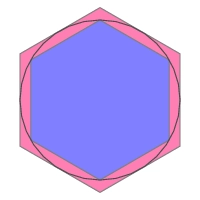# Difference 80618

A regular hexagon is described and inscribed in a circle. The difference between its areas is 8√3. Find the circle's radius.

r =  4

### Step-by-step explanation:Did you find an error or inaccuracy? Feel free to write us. Thank you!

Tips for related online calculators
The Pythagorean theorem is the base for the right triangle calculator.

#### You need to know the following knowledge to solve this word math problem:

We encourage you to watch this tutorial video on this math problem: